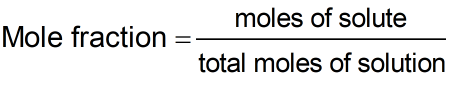Request a Tool

Mole Fraction Calculator

The ratio of the number of moles of one component of a solution

Solution
0
Fraction
0

FormulaDefination / Uses

The number of molecules (or moles) in one component divided by the total number of molecules (or moles) in the combination is referred to as the mole fraction. When two reactive components are combined together, mole fraction is important because the ratio of the two components may be calculated if the mole fraction of each component is known.

A mole is the number of fundamental units of reactant substances participating in a chemical reaction, while a fraction is the division of percentage of the reactant substances. As a result, the Mole Fraction equation can be defined as the ratio of reactant substances that react/combine to produce a product. The quickest approach to quickly determine mole fraction is to use a mole fraction calculator. Our mole fraction calculator is a useful tool that uses two ways to calculate the mole fraction of your solutions. It doesn't matter if you merely know how many moles are in a solution or if you have to work with solvents and solutes. Use the upper given formula for manual calculations. No sign-up, registration OR captcha is required to use this tool.